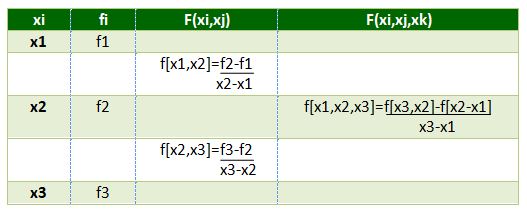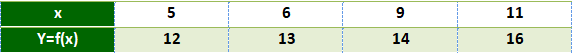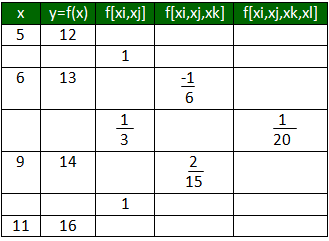# Newton’s Divided Difference Interpolation Formula

Interpolation is an estimation of a value within two known values in a sequence of values.

Newton’s divided difference interpolation formula is a interpolation technique used when the interval difference is not same for all sequence of values.

Suppose f(x0), f(x1), f(x2)………f(xn) be the (n+1) values of the function y=f(x) corresponding to the arguments x=x0, x1, x2…xn, where interval differences are not same
Then the first divided difference is given byThe second divided difference is given byand so on…
Divided differences are symmetric with respect to the arguments i.e independent of the order of arguments.
so,
f[x0, x1]=f[x1, x0]
f[x0, x1, x2]=f[x2, x1, x0]=f[x1, x2, x0]

By using first divided difference, second divided difference as so on .A table is formed which is called the divided difference table.

Divided difference table:NEWTON’S DIVIDED DIFFERENCE INTERPOLATION FORMULAExamples:

Input : Value at 7Output :Value at 7 is 13.47


## Recommended: Please try your approach on {IDE} first, before moving on to the solution.

Below is the implementation for Newton’s divided difference interpolation method.

## C++

 // CPP program for implementing  // Newton divided difference formula  #include  using namespace std;     // Function to find the product term  float proterm(int i, float value, float x[])  {      float pro = 1;      for (int j = 0; j < i; j++) {          pro = pro * (value - x[j]);      }      return pro;  }     // Function for calculating  // divided difference table  void dividedDiffTable(float x[], float y[], int n)  {      for (int i = 1; i < n; i++) {          for (int j = 0; j < n - i; j++) {              y[j][i] = (y[j][i - 1] - y[j + 1]                           [i - 1]) / (x[j] - x[i + j]);          }      }  }     // Function for applying Newton's  // divided difference formula  float applyFormula(float value, float x[],                     float y[], int n)  {      float sum = y;         for (int i = 1; i < n; i++) {        sum = sum + (proterm(i, value, x) * y[i]);      }      return sum;  }     // Function for displaying   // divided difference table  void printDiffTable(float y[],int n)  {      for (int i = 0; i < n; i++) {          for (int j = 0; j < n - i; j++) {              cout << setprecision(4) <<                                    y[i][j] << "\t ";          }          cout << "\n";      }  }     // Driver Function  int main()  {      // number of inputs given      int n = 4;      float value, sum, y;      float x[] = { 5, 6, 9, 11 };         // y[][] is used for divided difference      // table where y[] is used for input      y = 12;      y = 13;      y = 14;      y = 16;         // calculating divided difference table      dividedDiffTable(x, y, n);         // displaying divided difference table      printDiffTable(y,n);         // value to be interpolated      value = 7;         // printing the value      cout << "\nValue at " << value << " is "                << applyFormula(value, x, y, n) << endl;      return 0;  }

## Java

 // Java program for implementing  // Newton divided difference formula  import java.text.*;  import java.math.*;     class GFG{  // Function to find the product term  static float proterm(int i, float value, float x[])  {      float pro = 1;      for (int j = 0; j < i; j++) {          pro = pro * (value - x[j]);      }      return pro;  }     // Function for calculating  // divided difference table  static void dividedDiffTable(float x[], float y[][], int n)  {      for (int i = 1; i < n; i++) {          for (int j = 0; j < n - i; j++) {              y[j][i] = (y[j][i - 1] - y[j + 1]                          [i - 1]) / (x[j] - x[i + j]);          }      }  }     // Function for applying Newton's  // divided difference formula  static float applyFormula(float value, float x[],                  float y[][], int n)  {      float sum = y;         for (int i = 1; i < n; i++) {      sum = sum + (proterm(i, value, x) * y[i]);      }      return sum;  }     // Function for displaying   // divided difference table  static void printDiffTable(float y[][],int n)  {      DecimalFormat df = new DecimalFormat("#.####");      df.setRoundingMode(RoundingMode.HALF_UP);             for (int i = 0; i < n; i++) {          for (int j = 0; j < n - i; j++) {              String str1 = df.format(y[i][j]);              System.out.print(str1+"\t ");          }          System.out.println("");      }  }     // Driver Function  public static void main(String[] args)  {      // number of inputs given      int n = 4;      float value, sum;      float y[][]=new float;      float x[] = { 5, 6, 9, 11 };         // y[][] is used for divided difference      // table where y[] is used for input      y = 12;      y = 13;      y = 14;      y = 16;         // calculating divided difference table      dividedDiffTable(x, y, n);         // displaying divided difference table      printDiffTable(y,n);         // value to be interpolated      value = 7;         // printing the value      DecimalFormat df = new DecimalFormat("#.##");      df.setRoundingMode(RoundingMode.HALF_UP);             System.out.println("\nValue at "+df.format(value)+" is "             +df.format(applyFormula(value, x, y, n)));  }  }  // This code is contributed by mits

## Python3

 # Python3 program for implementing   # Newton divided difference formula      # Function to find the product term   def proterm(i, value, x):       pro = 1;       for j in range(i):           pro = pro * (value - x[j]);       return pro;      # Function for calculating   # divided difference table   def dividedDiffTable(x, y, n):         for i in range(1, n):           for j in range(n - i):               y[j][i] = ((y[j][i - 1] - y[j + 1][i - 1]) /                                      (x[j] - x[i + j]));      return y;     # Function for applying Newton's   # divided difference formula   def applyFormula(value, x, y, n):          sum = y;          for i in range(1, n):          sum = sum + (proterm(i, value, x) * y[i]);              return sum;      # Function for displaying divided   # difference table   def printDiffTable(y, n):          for i in range(n):           for j in range(n - i):               print(round(y[i][j], 4), "\t",                                  end = " ");              print("");      # Driver Code     # number of inputs given   n = 4;   y = [[0 for i in range(10)]           for j in range(10)];   x = [ 5, 6, 9, 11 ];      # y[][] is used for divided difference   # table where y[] is used for input   y = 12;   y = 13;   y = 14;   y = 16;      # calculating divided difference table   y=dividedDiffTable(x, y, n);      # displaying divided difference table   printDiffTable(y, n);      # value to be interpolated   value = 7;      # printing the value   print("\nValue at", value, "is",          round(applyFormula(value, x, y, n), 2))     # This code is contributed by mits

## C#

 // C# program for implementing   // Newton divided difference formula   using System;     class GFG{   // Function to find the product term   static float proterm(int i, float value, float[] x)   {       float pro = 1;       for (int j = 0; j < i; j++) {           pro = pro * (value - x[j]);       }       return pro;   }      // Function for calculating   // divided difference table   static void dividedDiffTable(float[] x, float[,] y, int n)   {       for (int i = 1; i < n; i++) {           for (int j = 0; j < n - i; j++) {               y[j,i] = (y[j,i - 1] - y[j + 1,i - 1]) / (x[j] - x[i + j]);           }       }   }      // Function for applying Newton's   // divided difference formula   static float applyFormula(float value, float[] x,                   float[,] y, int n)   {       float sum = y[0,0];          for (int i = 1; i < n; i++) {       sum = sum + (proterm(i, value, x) * y[0,i]);       }       return sum;   }      // Function for displaying   // divided difference table   static void printDiffTable(float[,] y,int n)   {       for (int i = 0; i < n; i++) {           for (int j = 0; j < n - i; j++) {               Console.Write(Math.Round(y[i,j],4)+"\t ");           }           Console.WriteLine("");       }   }      // Driver Function   public static void Main()   {       // number of inputs given       int n = 4;       float value;       float[,] y=new float[10,10];       float[] x = { 5, 6, 9, 11 };          // y[][] is used for divided difference       // table where y[] is used for input       y[0,0] = 12;       y[1,0] = 13;       y[2,0] = 14;       y[3,0] = 16;          // calculating divided difference table       dividedDiffTable(x, y, n);          // displaying divided difference table       printDiffTable(y,n);          // value to be interpolated       value = 7;          // printing the value              Console.WriteLine("\nValue at "+(value)+" is "             +Math.Round(applyFormula(value, x, y, n),2));   }   }   // This code is contributed by mits

## PHP

 

Output:

12     1     -0.1667     0.05
13     0.3333     0.1333
14     1
16

Value at 7 is 13.47


Attention reader! Don’t stop learning now. Get hold of all the important DSA concepts with the DSA Self Paced Course at a student-friendly price and become industry ready.

My Personal Notes arrow_drop_upCheck out this Author's contributed articles.

If you like GeeksforGeeks and would like to contribute, you can also write an article using contribute.geeksforgeeks.org or mail your article to contribute@geeksforgeeks.org. See your article appearing on the GeeksforGeeks main page and help other Geeks.

Please Improve this article if you find anything incorrect by clicking on the "Improve Article" button below.

Improved By : Mithun Kumar, akashagg